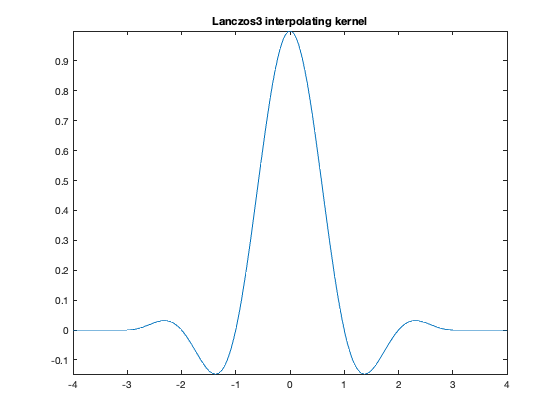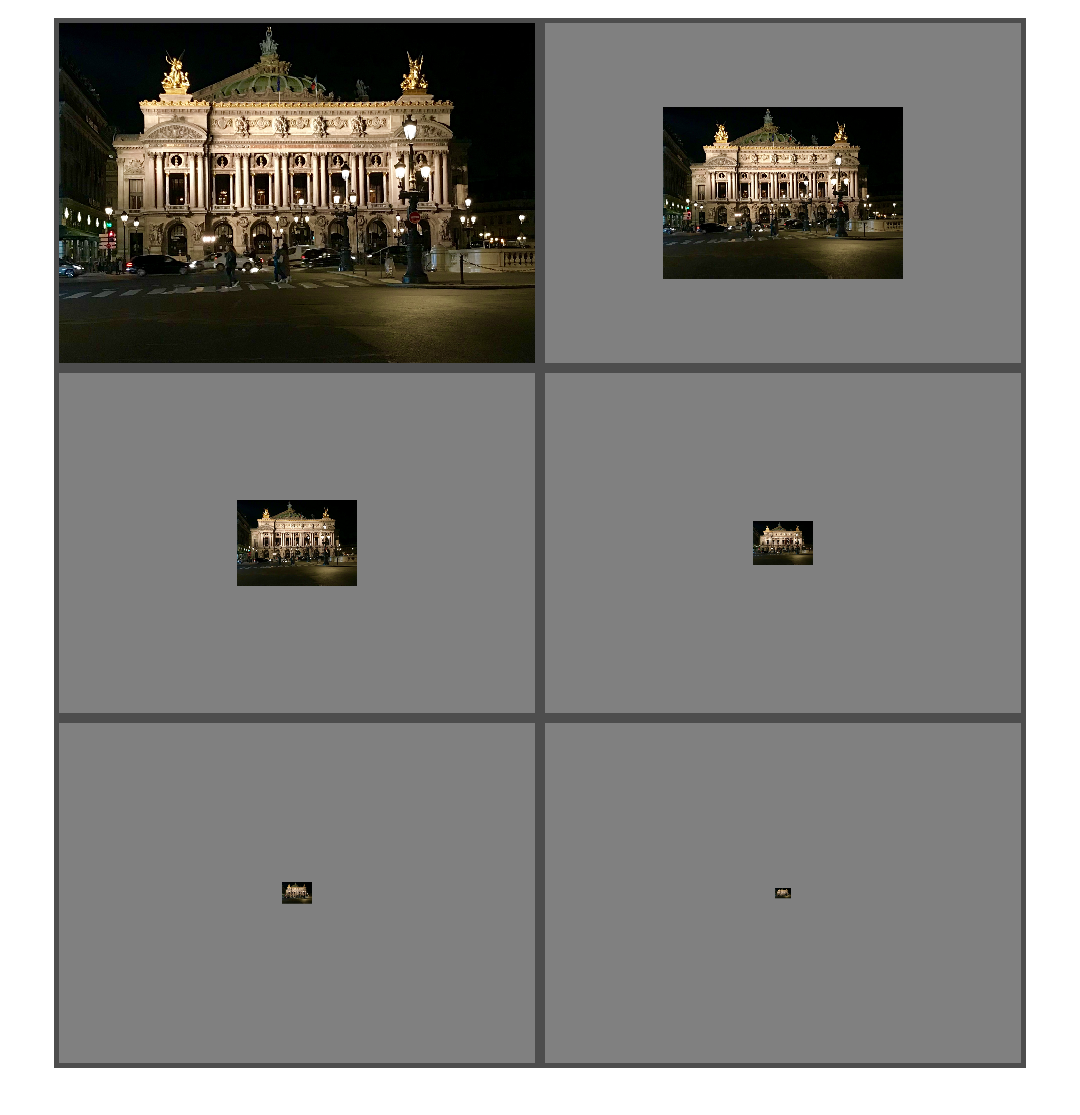# Multiresolution image pyramids and impyramid – part 2

Last time, I talked about the function impyramid and how I have been dissatisfied with it. (Confession: I designed it.) Today I want to present an alternative approach to creating a multiresolution pyramid.

Here are some of my wishes:

• Don't make me think about how many levels to specify. Find a good heuristic rule and apply it by default.
• Compute all the levels at once. Don't make me write a loop or initialize a place to keep the levels.
• Handle all the picky details like how to handle reducing a level with odd dimensions.
• Use a better resampling filter.
• Make the sizes match when you reduce a level and then expand it.

To start off, then, how many levels should we compute by default? Another way to ask that question is: how small do we want to let the last level get? Should we avoid letting the images get smaller than a certain size, such as 32x32? Or should we compute the levels all the way down to a scalar?

I don't know what is best. To answer the question, I would want to experiment with practical applications of multiresolution pyramids to find a heuristic rule that works well. For now, I'll go with a lower size limit of 32x32. Let's tinker with some code based on that rule.

A = imread('https://blogs.mathworks.com/steve/files/IMG_9968.jpg');
imshow(A)M = size(A,1);
N = size(A,2);
[M N]

ans =

1360        1904


lower_limit = 32;


Each level of the pyramid reduces the size by a factor of two, so the log2 function seems relevant.

log2([M N])

ans =

10.4094   10.8948


ans - log2(lower_limit)

ans =

5.4094    5.8948



If we don't divide by 2 more than the above number of times, then the smallest image size in the pyramid will be no smaller than lower_limit. Clearly, the number of size reduction steps has to be an integer.

num_levels = min(floor(log2([M N]) - log2(lower_limit)))

num_levels =

5



To handle degenerate cases smoothly, as well as to make visualizations easier, I want to include the original image as the first level in the pyramid, so ...

num_levels = num_levels + 1

num_levels =

6



Next, let's consider how to avoid odd image size dimensions when reducing each pyramid level. Our example image is 1360x1904, and if you just divide those numbers by 2 five times, you end up with [42.5 59.5]. Let's take the point of view that we want the smallest image in the pyramid to have an integer number of rows and columns. And then let's figure out how much to pad the original image so that we don't end up with any fractional rows and columns anywhere.

smallest_size = [M N] / 2^(num_levels - 1)

smallest_size =

42.5000   59.5000


smallest_size = ceil(smallest_size)

smallest_size =

43    60


padded_size = smallest_size * 2^(num_levels - 1)

padded_size =

1376        1920



I can imagine different ways to pad the original image, each of which has pros and cons. I'm inclined to do something simple, like pad to the right and bottom of the image by replicating the border pixels.

A_padded = padarray(A,padded_size - [M N],'replicate','post');


Now I need to pick a method for shrinking images by a factor of two to form each level. I propose to use imresize with the Lanczos3 interpolating kernel. (The lanczos3 function is at the bottom of this script.

figure
fplot(@lanczos3,[-4 4],'LineWidth',1.5)
title('Lanczos3 interpolating kernel')You can see that this is similar to a sinc function but nonzero only in the interval $|x| \leq 3$. The kernel has reasonably good sharpness and antialiasing behavior.

Now we're ready to shrink the original image (as padded) several times to form the multiresolution pyramid.

pyramid = cell(1,num_levels);
for k = 2:num_levels
pyramid{k} = imresize(pyramid{k-1},0.5,'lanczos3');
end


One final fix-up step: let's use the original (unpadded) image as level 1. One advantage of doing that is that we don't have to keep track of the original image size as a separate piece of data.

pyramid{1} = A;


The function multiresolutionPyramid, included at the bottom of this post, includes the logic and computations that I have described above. I have included another function, visualizePyramid, for visualizing the pyramid.

pyramid = multiresolutionPyramid(A);
visualizePyramid(pyramid)Next time, I expect to talk about Laplacian pyramids.

function f = lanczos3(x)
% See Graphics Gems, Andrew S. Glasser (ed), Morgan Kaufman, 1990,
% pp. 157-158.

f = (sin(pi*x) .* sin(pi*x/3) + eps) ./ ((pi^2 * x.^2 / 3) + eps);
f = f .* (abs(x) < 3);
end

function mrp = multiresolutionPyramid(A,num_levels)
%multiresolutionPyramid(A,numlevels)
%   mrp = multiresolutionPyramid(A,numlevels) returns a multiresolution
%   pyramd from the input image, A. The output, mrp, is a 1-by-numlevels
%   cell array. The first element of mrp, mrp{1}, is the input image.
%
%   If numlevels is not specified, then it is automatically computed to
%   keep the smallest level in the pyramid at least 32-by-32.

%   Steve Eddins
%   MathWorks

A = im2double(A);

M = size(A,1);
N = size(A,2);

if nargin < 2
lower_limit = 32;
num_levels = min(floor(log2([M N]) - log2(lower_limit))) + 1;
else
num_levels = min(num_levels, min(floor(log2([M N]))) + 2);
end

mrp = cell(1,num_levels);

smallest_size = [M N] / 2^(num_levels - 1);
smallest_size = ceil(smallest_size);
padded_size = smallest_size * 2^(num_levels - 1);

mrp{1} = Ap;
for k = 2:num_levels
mrp{k} = imresize(mrp{k-1},0.5,'lanczos3');
end

mrp{1} = A;
end

function tiles_out = visualizePyramid(p)
% Steve Eddins
% MathWorks

M = size(p{1},1);
N = size(p{1},2);

for k = 1:numel(p)
Mk = size(p{k},1);
Nk = size(p{k},2);

A = p{k};
p{k} = A;
end

tiles = imtile(p,'GridSize',[NaN 2],'BorderSize',20,'BackgroundColor',[0.3 0.3 0.3]);
imshow(tiles)

if nargout > 0
tiles_out = tiles;
end
end


Published with MATLAB® R2019a

|